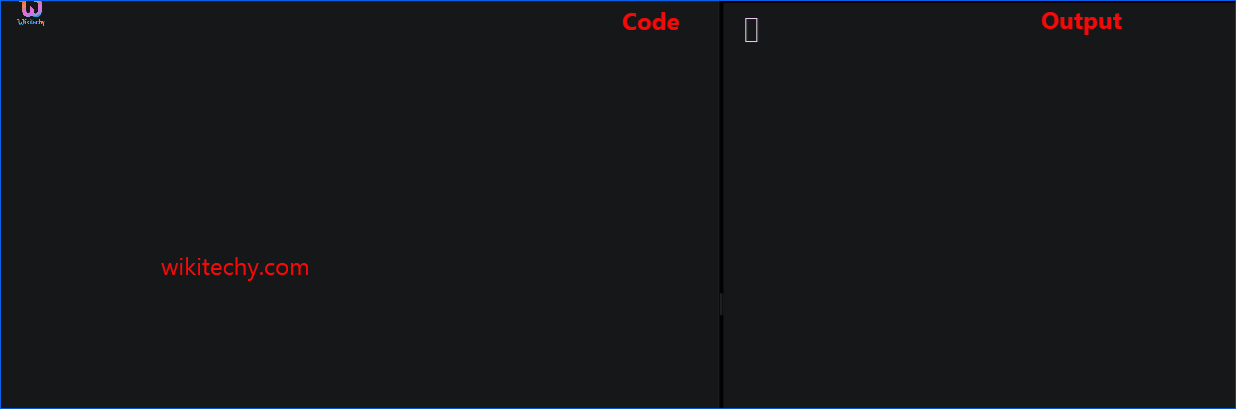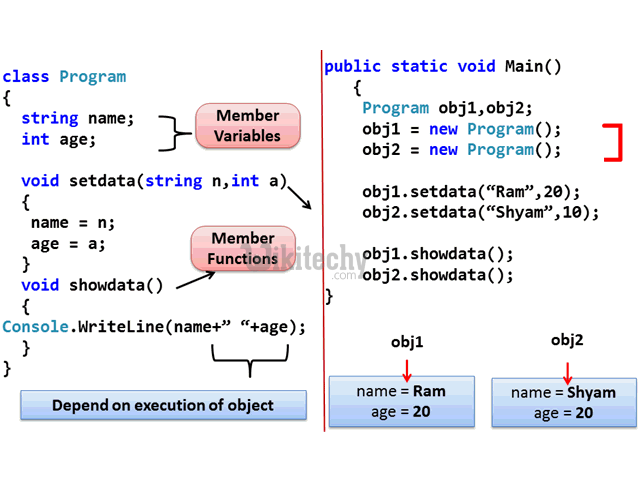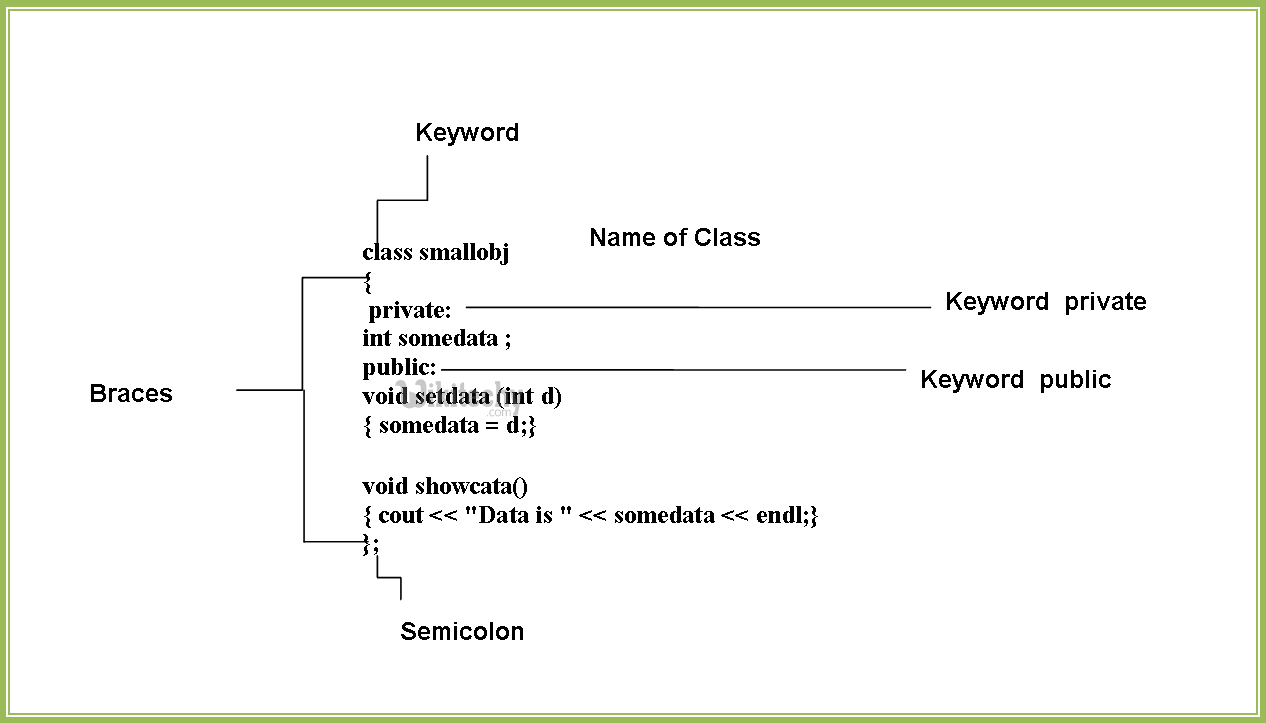# C++ Classes and Objects - Learn C++ - C++ Tutorial - C++ programmingLearn c++ - c++ tutorial - c++ classes and -objects - c++ examples - c++ programs

## C++ Class:

• Before you create an object in C++, you need to define a class.
• A class is a blueprint for the object.
•learn c++ tutorials - class object model in c++ Example

• We can think of class as a sketch (prototype) of a house. It contains all the details about the floors, doors, windows etc. Based on these descriptions we build the house. House is the object.
• As, many houses can be made from the same description, we can create many objects from a class.
•learn c++ tutorials - class and object in c++ Example

## How to define a class in C++?

• A class is defined in C++ using keyword class followed by the name of class.
• The body of class is defined inside the curly brackets and terminated by a semicolon at the end.

## Example: Class in C++

• Here, we defined a class named Test.
• This class has two data members: data1 and data2 and two member functions:function1() and function2().

## Keywords: private and public

• You may have noticed two keywords: private and public in the above example.
• The private keyword makes data and functions private. Private data and functions can be accessed only from inside the same class.
• The public keyword makes data and functions public. Public data and functions can be accessed out of the class.
• Here, data1 and data2 are private members where as function1() and function2() are public members.
•learn c++ tutorials - class and object definition in c++ Example

• If you try to access private data from outside of the class, compiler throws error. This feature in OOP is known as data hiding.

## C++ Objects

• When class is defined, only the specification for the object is defined; no memory or storage is allocated.
• To use the data and access functions defined in the class, you need to create objects.

## Syntax to Define Object in C++

• You can create objects of Test class (defined in above example) as follows:
• Here, two objects o1 and o2 of Test class are created.
• In the above class Test, data1 and data2 are data members and function1() andfunction2() are member functions.

## How to access data member and member function in C++?

• You can access the data members and member functions by using a . (dot) operator. For example,
• This will call the function1() function inside the Test class for objects o2.
• Similarly, the data member can be accessed as:
• It is important to note that, the private members can be accessed only from inside the class.
• So, you can use o2.function1(); from any function or class in the above example. However, the code o1.data2 = 5.5; should always be inside the class Test.

## Output

• In this program, two data members data1 and data2 and two member functions insertIntegerData() and insertFloatData() are defined under Test class.
• Two objects o1 and o2 of the same class are declared.
• The insertIntegerData() function is called for the o1 object using:
• This sets the value of data1 for object o1 to 12.
• Then, the insertFloatData() function for object o2 is called and the return value from the function is stored in variable secondDataOfObject2 using:
• In this program, data2 of o1 and data1 of o2 are not used and contains garbage value.